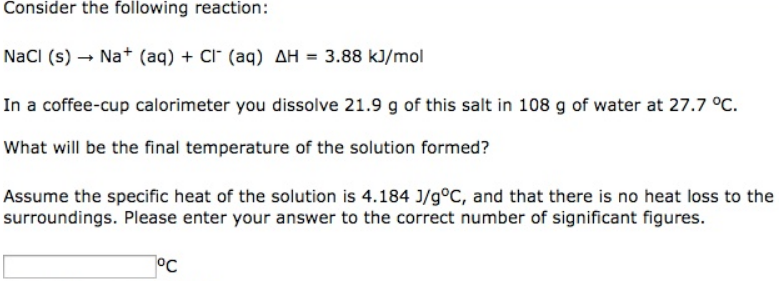# Problem: Consider the following reaction: NaCl (s) → Na+ (aq) + Cl- (aq)         ΔH = 3.88 kJ/mol In a coffee-cup calorimeter you dissolve 21.9 g of this salt in 108 g of water at 27.7°C. What will be the final temperature of the solution formed? Assume the specific heat of the solution is 4.184 J/g°C, and that there is no heat loss to the surroundings. Please enter your answer to the correct number of significant figures.

###### FREE Expert Solution
86% (460 ratings)###### Problem Details

Consider the following reaction:

NaCl (s) → Na+ (aq) + Cl- (aq)         ΔH = 3.88 kJ/mol

In a coffee-cup calorimeter you dissolve 21.9 g of this salt in 108 g of water at 27.7°C.

What will be the final temperature of the solution formed?

Assume the specific heat of the solution is 4.184 J/g°C, and that there is no heat loss to the surroundings. Please enter your answer to the correct number of significant figures.